# Java Program to print sum of all the items of the array

In this tutorial you will learn about the Java Program to print sum of all the items of the array and its application with practical example.

In this tutorial, we will learn to create a Java Program to print sum of all the items of the array  using Java programming.

## Prerequisites

Before starting with this tutorial, we assume that you are the best aware of the following Java programming topics:

• Java Operators.
• Basic Input and Output function in Java.
• Class and Object in Java.
• Basic Java programming.
• If-else statements in Java.
• For loop in Java.

## What is An array?

The array is a collection of similar data types. An array can store multiple values with different indexes in memory by using a single variable. The array can be both single and multidimensional.

## Program description to Find Sum of all Elements in an Array.

In this program, we will first take the input array size and the elements from the user. Then we will add all the elements of the array using the for loop. At last, we will print that sum of elements of the array using the print function.

## Output:-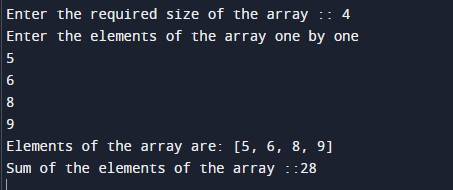In the above program, we have first initialized the required variable.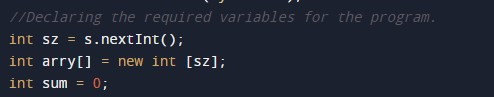• sum = it will hold the integer value for the sum of numbers.
• arry[] = it will hold the integer value of the input elements.
• i = it will hold the integer value.
• sz = it will hold the integer value of the input.

Input the number of elements and the elements of the array from the user.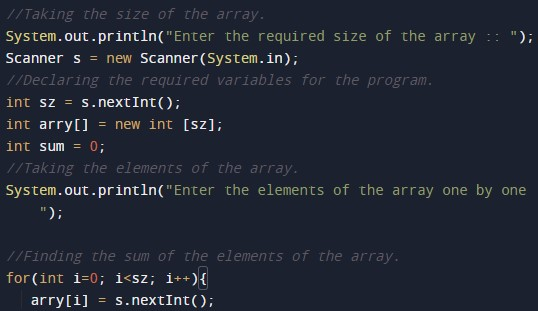Program Logic Code to find the sum of an array.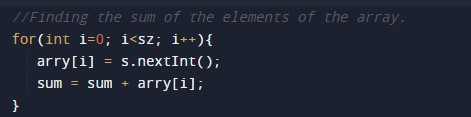Printing output sum of the array elements.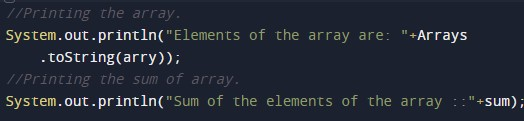In this tutorial we have learn about the Java Program to print sum of all the items of the array and its application with practical example. I hope you will like this tutorial.